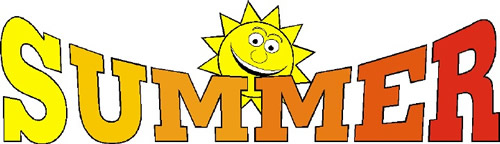•
Thank you students for an awesome 2021-2022 school year full of math and science.  Here are some key concepts we learned and completed during your 4th grade experience:

1)  Understanding place value up to millions place
2)  Addition/Subtraction with regrouping up to millions place
3)  Multiplication and Division up to 4 digits by 1 digit for multiplication, and 4 digit dividend
4)  Fractions:
a)  Finding and understanding equivalence
b)  adding and subtracting like and unlike denominators
c)  comparing & ordering fractions on a number line
d)  Decomposing mixed numbers and converting them into improper fractions
e)  Multiplying a fraction times a whole number
f)   Converting a fraction to a decimal
5)  Decimal
a)  Understanding tenths and hundredths
b)  Drawing models comparing tenths & hundreds
6)   Geometry:
a)   Parallel & Perpendicular
b)  Angles
1)  Measuring using a protractor
2)  Identfiy angles

Please feel free to continue practicing these concepts during the summer and focus on the following three for entering into 5th grade:
1)   Power of 10
2)  Round numbers to any place up to a thousandth place
3)  Multiply and Divide using a double digit divisor

Be safe, enjoy your time by playing with friends and family, and come back with your best summer story or experience.

Mrs. Rawls
4th Grade/Math & Science Teacher.
email:  rawlsjam@orange.k12.nj.us